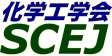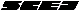## \$B9V1i%W%m%0%i%`!J2q>l!&F|DxJL!K(B

### H\$B2q>l(B \$BBh(B2\$BF|(B

\$B:G=*99?7F|;~!'(B2012-08-20 15:02:17
\$B9V1i(B
\$B;~9o(B
\$B9V1i(B
\$BHV9f(B
\$B9V1iBjL\!?H/I=\$B%-!<%o!<%I(B\$BJ,N`(B
\$BHV9f(B
\$B\$BHV9f(B
\$B%7%s%]%8%&%`(B \$B!c;q8;!&%(%M%k%.!
(9:00\$B!A(B10:00)\$B!!(B(\$B:BD9(B \$BB?8P(B \$B516=(B)
9:00\$B!A(B 9:20H201\$B79l\$K\$*\$1\$k9|3J0[@-2=\$NB%?J(B
(\$B \$B!&(B (\$B3X(B)\$B?"ED(B \$BLw9((B \$B!&(B (\$B@5(B)\$B?eED(B \$B7I(B
FCC
Skeltal isomerization
S-5984
9:20\$B!A(B 9:40H202\$B%P%\$%*%^%9\$+\$iM6F3\$5\$l\$k4^;@AG2=9gJ*\$N%<%*%i%\$%H\$K\$h\$k2=3X86NA2=(B
(\$B \$B!&(B (\$B3X(B)\$BEl(B \$BC#Lo(B \$B!&(B (\$B3X(B)\$BCSED(B \$B7=M\$(B \$B!&(B (\$B@5(B)\$B?eED(B \$B7I(B
Biomass
Zeolite
Chemicals
S-5990
9:40\$B!A(B 10:00H203\$B%j%s;@=hM}(BH-ZSM-5\$B\$K\$h\$k%a%?%N!<%k\$+\$i\$N%W%m%T%l%s9g@.(B
(\$B:eBg1!4p9)(B) \$B!{(B(\$B3X(B)\$BCfLn(B \$BM\$0l(B \$B!&(B (\$BEl9)Bg1!M}9)(B) (\$B@5(B)\$BW"ED(B \$BM:0lO/(B \$B!&(B (\$B:eBg1!4p9)(B) (\$B@5(B)\$BFbED(B \$B9,L@(B \$B!&(B (\$B@5(B)\$B9>F,(B \$BLw9,(B \$B!&(B (\$BEl%=!<(B) \$B5HED(B \$BCR(B \$B!&(B (\$B:eBg1!4p9)(B) (\$B@5(B)\$B@>;3(B \$B7{OB(B
Light olefn
Methanol-to-olefn
MFI
S-5622
(10:00\$B!A(B11:00)\$B!!(B(\$B:BD9(B \$B@>;3(B \$B7{OB(B)
10:00\$B!A(B 10:20H204n-\$B%X%-%5%s@\?(J,2rH?1~\$GF@\$i\$l\$?(BCoke\$BIUCe(BZSM-5\$B\$NG3>F:F@8H?1~B.EY2r@O(B
(\$BKLBg1!9)(B) \$B!{(B(\$B@5(B)\$BCf:d(B \$BM\$B@(B \$B!&(B \$B:#Ln(B \$BBg51(B \$B!&(B (\$B;00f2=3X(B) \$B2,It(B \$B98Gn(B \$B!&(B (\$BKLBg1!9)(B) (\$B@5(B)\$BB?8P(B \$B516=(B \$B!&(B (\$B@5(B)\$BA}ED(B \$BN4IW(B
Regeneration
Coke-ZSM-5
Simulation
S-5492
10:20\$B!A(B 10:40H205\$B%<%*%i%\$%H?(G^>e\$G\$N4D>u%1%H%s\$N(BBaeyer-Villiger\$B;@2=H?1~\$K\$*\$1\$k%<%*%i%\$%H9=B\$\$N1F6A(B
(\$B?@8MBg1!9)(B) \$B!{(B(\$B3X(B)\$BCv86(B \$B90BY(B \$B!&(B (\$B3X(B)\$BCf0f(B \$B9/M5(B \$B!&(B \$BFbF#(B \$B???M(B \$B!&(B (\$B@5(B)\$B;T66(B \$BM40l(B \$B!&(B (\$B@5(B)\$B@>;3(B \$B3P(B
zeolite structure
Baeyer-Villiger
cyclic ketones
S-5670
10:40\$B!A(B 11:00H206\$BI9>=%F%s%W%l!<%HK!\$rMQ\$\$\$?%^%\$%/%m%O%K%+%`>u
(\$BKLBg1!9)(B) \$B!{(B(\$B3X(B)\$BKeHx(B \$B854p(B \$B!&(B (\$B3X(B)\$B:4F#(B \$B7D9'(B \$B!&(B (\$B@5(B)\$BB<>e(B \$B0lGn(B \$B!&(B (\$B@5(B)\$B2.Ln(B \$B7.(B \$B!&(B (\$B@5(B)\$B8~0f(B \$B?B(B
ice templating method
ion exchange resin
microhoneycomb
S-5551
(11:00\$B!A(B12:00)\$B!!(B(\$B:BD9(B \$B@>;3(B \$B3P(B)
11:00\$B!A(B 11:20H207\$BL55!%(%"%m%>%k?eOBJ*\$NF1Dj\$H5[<>@-I>2A(B
(\$B72Bg1!(B) \$B@P:d(B \$BLw;V(B \$B!&(B \$B!{(B(\$B@5(B)\$B86Ln(B \$B0BEZ(B
hydrate
hygroscopicity
Raman Spectroscopy
S-5651
11:20\$B!A(B 11:40H208\$B?(G^\$rMQ\$\$\$?%"%s%b%K%"J,2r\$K4X\$9\$k8&5f(B
(\$BElG@9)Bg1!9)(B) \$B!{(B(\$B3X(B)\$B1JEg(B \$B?pJf(B \$B!&(B (\$B@5(B)\$B55;3(B \$B=(M:(B \$B!&(B (\$B@5(B)\$B:y0f(B \$B@?(B
ammonia
catalyst
decomposition
S-5393
11:40\$B!A(B 12:00H209\$B%0%j%;%j%s\$r86NA\$H\$7\$?(B1,3-\$B%W%m%Q%s%8%*!<%k9g@.%W%m%;%9(B
(\$BF|N)(B) \$B!{(B(\$B@5(B)\$B>e@n(B \$B>-9T(B \$B!&(B (\$B@5(B)\$B6aF#(B \$B7rG7(B \$B!&(B (\$B@5(B)\$B>>Hx(B \$B=SL@(B \$B!&(B (HPT) \$B:4@\$(B \$B9/@.(B \$B!&(B \$BC0F#(B \$B=g;V(B
1,3-propanediol
glycerine
PTT
S-5660
(13:00\$B!A(B14:20)\$B!!(B(\$B:BD9(B \$B4X:,(B \$BBY(B)
13:00\$B!A(B 13:20H213\$B%"%k%_%JC4;}%K%C%1%k?(G^\$K\$*\$1\$k%K%C%1%k\$N4T85FC@-\$H?(G^H?1~FC@-(B
(\$B6eBg1!9)(B) \$B!{(B(\$B3X(B)\$BKv5H(B \$BKc0a(B \$B!&(B (\$B@5(B)\$B>>:,(B \$B1Q \$B!&(B (\$B@5(B)\$BC]Cf(B \$BAT(B \$B!&(B (\$B@5(B)\$B4_ED(B \$B>;9@(B
Ni catalyst
partial oxidation of methane
reduction characteristic
S-5623
13:20\$B!A(B 13:40H214Aromatic Hydrocarbon Steam Reforming Using Surface Oxidized Hastelloy as Catalyst
(\$BL>Bg1!9)(B) \$B!{(B(\$B3X(B)de la Rama Sharon Rose \$B!&(B (\$B3X(B)\$B2O0f(B \$B?5B@O:(B \$B!&(B (\$B@5(B)\$B;3ED(B \$BGn;K(B \$B!&(B (\$B@5(B)\$BED@n(B \$BCRI'(B
steam reforming
aromatic hydrocarbon
hastelloy
S-5626
13:40\$B!A(B 14:00H215\$BDc29?e@-%,%9%7%U%H\$N\$?\$a\$N(BCu-Zn-Al\$B?(G^\$N@-G=Nt2=\$KM?\$(\$k6u4VB.EY\$N1F6A(B
(\$B9)3X1!Bg9)(B) \$B!{(B(\$B3X(B)\$BD90f(B \$B0l5.(B \$B!&(B (\$B@5(B)\$BHSED(B \$BH%(B \$B!&(B (\$B@5(B)\$B8^==Mr(B \$BE/(B
Water Gas Shift
Cu-Zn-Al Catalyst
Deactivation
S-5647
14:00\$B!A(B 14:20H216\$B%a%?%k%O%K%+%`7?(BNi\$B7O9=B\$BN?(G^\$K\$h\$k%a%?%s\$ND>@\J,2rH?1~(B
(\$B@EBg9)(B) \$B!{(B(\$B@5(B)\$BEOIt(B \$B0=(B \$B!&(B (\$B@EBg1!(B) (\$B3X(B)\$BJ \$B!&(B (\$B3X(B)\$B?yK\(B \$B980l(B \$B!&(B (\$B@EBg9)(B) (\$B@5(B)\$BJ!86(B \$BD9
Structured catalyst
Methane decomposition
Durability
S-5230
(14:20\$B!A(B15:20)\$B!!(B(\$B:BD9(B \$B4_ED(B \$B>;9@(B)
14:20\$B!A(B 14:40H217Pd\$BKl7?H?1~4o\$rMQ\$\$\$?%P%\$%*%(%?%N!<%k\$N?e>x5\$2~r7o\$N8!F\$(B
(\$BFAEgBg1!@hC<650iIt(B) \$B!{(B(\$B3X(B)\$BLZFb(B \$B9@Fs(B \$B!&(B \$B3@Fb(B \$BC#Li(B \$B!&(B (\$B3X(B)\$B@P0f(B \$B0!M}:;(B \$B!&(B \$BKL86(B \$BM'N<(B \$B!&(B (\$BFAEgBg1!(BSTS\$B8&5fIt(B) (\$B@5(B)\$B2CF#(B \$B2mM5(B \$B!&(B (\$B@5(B)\$BKY2O(B \$B=S1Q(B \$B!&(B (\$B@5(B)\$BCf@n(B \$B7I;0(B \$B!&(B (\$B@5(B)\$B?y;3(B \$BLP(B \$B!&(B (\$B;0OBECJ49)6H(B) \$B5H@n(B \$BBn;V(B \$B!&(B \$BOBED(B \$B
Bio-ethanol
Hydrogen production
S-5333
14:40\$B!A(B 15:00H218\$B?eAG2=C&N2!&C&CbAGH?1~\$K\$*\$1\$k(BNiMoP/Al2O3\$BN22=?(G^\$N3h@-E@I>2A(B
(\$BElG@9)Bg(B) Nguen Thanh Tung \$B!&(B \$B?{0f(B \$BBg5.(B \$B!&(B \$BIZ1J(B \$B90G7(B \$B!&(B \$B!{(B(\$B@5(B)\$B1J0f(B \$B@5IR(B
Hydrodesulfurization
Hydrodenitrogenation
NiMo on alumina
S-5921
15:00\$B!A(B 15:20H219\$B%0%i%U%!%\$%H!&%0%i%U%'%s;@2=J*?(G^\$K\$h\$k%U%'%N!<%k\$N=-AG2=\$*\$h\$S%"%k%-%k2=H?1~A*Br@-\$HI=LLFC@-(B
(\$BElG@9)Bg(B) \$B@P9u(B \$B9'B@O/(B \$B!&(B \$B0k9>(B \$BN \$B!&(B \$B@6?e(B \$B>;;R(B \$B!&(B \$B@iMU(B \$B6A(B \$B!&(B \$BIZ1J(B \$B90G7(B \$B!&(B \$B!{(B(\$B@5(B)\$B1J0f(B \$B@5IR(B
Graphite oxide
Bromination
Graphene oxide
S-5881

\$B9V1i%W%m%0%i%`(B
\$B2=3X9)3X2q(B \$BBh(B44\$B2s=)5(Bg2q(B(C) 2012 \$B8x1W
Most recent update: 2012-08-20 15:02:17
For more information contact \$B2=3X9)3X2qElKL;YIt(B \$BBh(B44\$B2s=)5(Bg2q(B \$BLd\$\$9g\$;78(B
E-mail: inquiry-44fwww3.scej.org
This page was generated byeasp 2.28; proghtml 2.28c (C)1999-2011 kawase